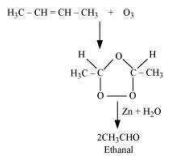Question 13.6:- An alkene ‘A’ contains three C – C, eight C – H σ bonds, and one C – C π bond. ‘A’ on ozonolysis gives two moles of an aldehyde of molar mass 44 u. Write the IUPAC name of ‘A’.

As per the given information, ‘A’ on ozonolysis gives two moles of an aldehyde of molar mass 44 u. The formation of two moles of an aldehyde indicates the presence of identical structural units on both sides of the double bond containing carbon atoms. Hence, the structure of ‘A’ can be represented as:
XC = CX

There are eight C–H σ bonds. Hence, there are 8 hydrogen atoms in ‘A’. Also, there are three C–C bonds. Hence, there are four carbon atoms present in the structure of ‘A’.Combining the inferences, the structure of ‘A’ can be represented as:


‘A’ has 3 C–C bonds, 8 C–H σ bonds, and one C–C π bond.
Hence, the IUPAC name of ‘A’ is But-2-ene.
Ozonolysis of ‘A’ takes place as:The final product is ethanal with a molecular mass